Theory and Modern Applications

# Exponential stabilization of uncertain nonlinear time-delay systems

## Abstract

This paper is concerned with the problem of exponential stabilization for a class of uncertain nonlinear systems with state delay by means of periodically intermittent control. Based on the Lyapunov-Krasovskii functional approach, several sufficient conditions of exponential stabilization for this class of uncertain nonlinear systems with state delay are proposed, which have been expressed in terms of linear matrix inequalities (LMIs) whose feasibility can be easily checked by using the numerically efficient Matlab LMI Toolbox. Further, the control design method is extended to a class of nonlinear systems with state delay. And the new stability criterion is also presented, which guarantees the closed-loop systems are exponentially stable. Finally, two numerical examples are given to show the effectiveness of the proposed approach.

MSC:93D15, 93C55, 34K20, 34D23.

## 1 Introduction

Time delay naturally appears in many control systems and is frequently a source of instability . In recent years, the problem of stability and stabilization of time-delay systems is a topic of great practical importance, which has attracted a lot of interest [14, 1020]. In , in the light of the Razumikhin stability theorem combined with the Newton-Leibniz formula, a new delay-dependent exponential stability condition was derived for linear non-autonomous time-delay systems. In , the sufficient conditions for absolute stability of nonlinear control systems of neutral type were obtained, and the estimates of exponential damping of solutions were constructed. In , the robust controller design for uncertain input-delayed systems was considered, and the stability criterion of the closed-loop system was derived. In , stability results of quadratic discrete systems in the critical case were presented, and the stability domains were estimated. In , an improved delay-dependent stability condition for a system with multiple additive delay components was derived by using the Lyapunov second method. In , the sufficient conditions for global asymptotic stabilization of nonlinear systems were given, and the corresponding feedback control laws were designed. In , the problem of quadratic stabilization of multi-input multi-output switched nonlinear systems under an arbitrary switching law was investigated. The state feedbacks were designed, and a common quadratic Lyapunov function of all the closed-loop subsystems was constructed to realize quadratic stabilization of the class of switched nonlinear systems under an arbitrary switching law.

Recently, in-continuous control techniques such as impulsive control  and piecewise feedback control  have attracted much attention. In , impulsive control for master-slave synchronization schemes that consist of identical chaotic Lur’e systems was considered. Impulsive control laws which made use of linear static measurement feedback instead of full state feedback were investigated. The recent paper  studied the output regulation problem for a class of discrete-time nonlinear systems under periodic disturbances generated from the so-called exosystems. By exploiting the structural information encoded in the fuzzy rules, a piecewise state-feedback and a piecewise error-feedback control laws were constructed to achieve asymptotic rejecting of the unwanted disturbances and/or tracking of the desired trajectories.

In this paper, we investigate the problem of exponential stabilization for a class of uncertain nonlinear systems by using another in-continuous feedback, i.e., periodically intermittent control. Intermittent control is a special form of switching control . It has been used for a variety of purposes in engineering fields such as manufacturing, transportation, air-quality control, communication and so on. Recently, intermittent control has been introduced to chaotic dynamical systems .  described the method of synchronizing slave to the master trajectory using intermittent coupling. However,  gave little theoretical analysis for intermittent control systems, but only many numerical simulations. In , the authors investigated the exponential stabilization problem for a class of chaotic systems with delay by means of periodically intermittent control. In , the quasi-synchronization problem for chaotic neural networks with parameter mismatch was formulated via periodically intermittent control.

Motivated by the aforementioned discussion, in this paper, we study a class of intermittent control with time duration for a class of uncertain nonlinear time-delay systems. Namely, the control is activated in certain nonzero time intervals, and it is off in other time intervals. Based on the Lyapunov-Krasovskii functional approach, exponential stability criteria for the class of uncertain nonlinear time-delay systems are given, which are presented in terms of linear matrix inequalities (LMIs). Moreover, this approach of control design has been generalized to a class of nonlinear systems with state delay. The new exponentially stabilization criterion for this class of nonlinear time-delay systems is proposed. Finally, two numerical examples are given to demonstrate the validity of the result.

The rest of this paper is organized as follows. In Section 2, the intermittent control problem is formulated and some notations and lemmas are introduced. In Section 3, the exponential stabilization problem for a class of uncertain nonlinear time-delay systems is investigated by means of periodically intermittent control, and some exponential stability criterions are established. Furthermore, the exponential stabilization method is expanded to a class of nonlinear systems with state delay. Two numerical examples are presented in Section 4. Finally, some conclusions and remarks are drawn in Section 5.

## 2 Problem formulation and preliminaries

Consider a class of nonlinear systems with delayed state described by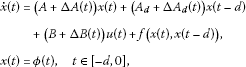(2.1)

where $x\in {R}^{n}$ is a state vector, and $u\in {R}^{m}$ is the external input of the system (2.1). $f:{R}^{n}\to {R}^{n}$ is a continuous nonlinear function with $f\left(0,0\right)=0$, and there exist positive definite matrices ${Q}_{1}$, ${Q}_{2}$ such that ${\parallel f\left(x\left(t\right),x\left(t-d\right)\right)\parallel }^{2}\le {x}^{T}\left(t\right){Q}_{1}x\left(t\right)+{x}^{T}\left(t-d\right){Q}_{2}x\left(t-d\right)$ for $x\left(t\right),x\left(t-d\right)\in {R}^{n}$. The initial function $\varphi \left(t\right)\in C\left(\left[-d,0\right],{R}^{n}\right)$, $d>0$, has its norm $\parallel \varphi \parallel ={sup}_{s\in \left[-d,0\right]}\parallel \varphi \left(s\right)\parallel$. $\mathrm{\Delta }A\left(t\right)$, $\mathrm{\Delta }{A}_{d}\left(t\right)$ and $\mathrm{\Delta }B\left(t\right)$ are time-varying uncertainties, which satisfy the following conditions:

$\mathrm{\Delta }A\left(t\right)={D}_{1}F\left(t\right){E}_{1},\phantom{\rule{2em}{0ex}}\mathrm{\Delta }{A}_{d}\left(t\right)={D}_{2}F\left(t\right){E}_{2},\phantom{\rule{2em}{0ex}}\mathrm{\Delta }B\left(t\right)={D}_{3}F\left(t\right){E}_{3},$
(2.2)

where ${D}_{i}$, ${E}_{i}$, $i=1,2,3$, are real constant matrices of appropriate dimensions, and $F\left(t\right)$ is an unknown time-varying matrix with ${F}^{T}\left(t\right)F\left(t\right)\le I$.

The following lemmas are useful in the proof of our main results.

Lemma 2.1 ()

Let D, E and F be real matrices of appropriate dimensions with ${F}^{T}F\le I$, then for any scalar $\epsilon >0$, we have the following inequality:

$DFE+{E}^{T}{F}^{T}{D}^{T}\le {\epsilon }^{-1}D{D}^{T}+\epsilon {E}^{T}E.$

Lemma 2.2 ()

Let M, N be real matrices of appropriate dimensions. Then for any matrix $Q>0$ of appropriate dimension and any scalar $\beta >0$, the following inequality holds:

$MN+{N}^{T}{M}^{T}\le {\beta }^{-1}M{Q}^{-1}{M}^{T}+\beta {N}^{T}QN.$

Lemma 2.3 ()

Given constant matrices ${S}_{1}$, ${S}_{2}$ and ${S}_{3}$ with appropriate dimensions, where ${S}_{1}={S}_{1}^{T}$, and $0<{S}_{3}={S}_{3}^{T}$, then ${S}_{1}+{S}_{2}{S}_{3}^{-1}{S}_{2}^{T}<0$ if and only if

$\left[\begin{array}{cc}{S}_{1}& {S}_{2}\\ {S}_{2}^{T}& -{S}_{3}\end{array}\right]<0.$

In order to stabilize the system (2.1) by means of periodically intermittent feedback control, we assume that the control imposed on the system is of the form

$u\left(t\right)=\left\{\begin{array}{cc}Kx\left(t\right),\hfill & nT\le t
(2.3)

where $K\in {R}^{m×n}$ is the control gain matrix, $T>0$ denotes the control period, and $\tau >0$ is called the control width.

With the control law (2.3), the system (2.1) can be rewritten as

$\left\{\begin{array}{c}\stackrel{˙}{x}\left(t\right)=\left(A+\mathrm{\Delta }A\left(t\right)\right)x\left(t\right)+\left({A}_{d}+\mathrm{\Delta }{A}_{d}\left(t\right)\right)x\left(t-d\right)\hfill \\ \phantom{\stackrel{˙}{x}\left(t\right)=}+\left(B+\mathrm{\Delta }B\left(t\right)\right)Kx\left(t\right)+f\left(x\left(t\right),x\left(t-d\right)\right),\hfill & nT\le t
(2.4)

This is a classical uncertain switched system with delayed state where the switching rule only depends on the time. Although there are many successful applications of intermittent control, the theoretical analysis of an intermittent control system has received little attention. In this brief, we will make a contribution to this issue.

Throughout this brief, we use ${P}^{T}$, ${\lambda }_{min}\left(P\right)$ (${\lambda }_{max}\left(P\right)$) to denote the transpose and the minimum (maximum) eigenvalue of a square matrix P, respectively. The vector (or matrix) norm is taken to be Euclidean, denoted by $\parallel \cdot \parallel$. We use $P>0$ (<0, ≤0, ≥0) to denote a positive (negative, semi-negative, semi-positive) definite matrix P. The symmetric term in a symmetric matrix is denoted as .

## 3 Exponential stabilization of a class of nonlinear systems with time-delay

This section addresses the exponential stability problem of the switched system (2.4). The main result is stated as follows.

Theorem 3.1 If there exist positive definite matrices $P>0$, $Q>0$ and scalar constants ${\epsilon }_{ij}>0$ ($i=1,2$, $j=1,2,3$), ${\epsilon }_{14}>0$, $w>0$, such that the following matrix inequalities hold: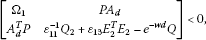(3.1)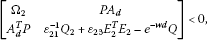(3.2)

where

$\begin{array}{rcl}{\mathrm{\Omega }}_{1}& =& {A}^{T}P+PA+{K}^{T}{B}^{T}P+PBK+wP+Q+{\epsilon }_{11}PP+{\epsilon }_{11}^{-1}{Q}_{1}+{\epsilon }_{12}^{-1}{E}_{1}^{T}{E}_{1}+{\epsilon }_{12}P{D}_{1}{D}_{1}^{T}P\\ +{\epsilon }_{14}^{-1}{K}^{T}{E}_{3}^{T}{E}_{3}K+{\epsilon }_{14}P{D}_{3}{D}_{3}^{T}P+{\epsilon }_{13}^{-1}P{D}_{2}{D}_{2}^{T}P,\end{array}$

and

${\mathrm{\Omega }}_{2}={A}^{T}P+PA+wP+Q+{\epsilon }_{21}PP+{\epsilon }_{21}^{-1}{Q}_{1}+{\epsilon }_{22}^{-1}{E}_{1}^{T}{E}_{1}+{\epsilon }_{22}P{D}_{1}{D}_{1}^{T}P+{\epsilon }_{23}^{-1}P{D}_{2}{D}_{2}^{T}P,$

then the system (2.4) is exponentially stable. Moreover, the solution $x\left(t\right)$ satisfies the condition

$\parallel x\left(t\right)\parallel \le \parallel \varphi \parallel \sqrt{\frac{{\lambda }_{max}\left(P\right)+d{\lambda }_{max}\left(Q\right)}{{\lambda }_{min}\left(P\right)}}{e}^{-\frac{w\left(t-\tau \right)}{2}},\phantom{\rule{1em}{0ex}}\mathrm{\forall }t>0.$

Proof Consider the following candidate Lyapunov-Krasovskii functional:

$V\left(x\left(t\right)\right)={x}^{T}\left(t\right)Px\left(t\right)+{e}^{-wt}{\int }_{t-d}^{t}{e}^{w\theta }{x}^{T}\left(\theta \right)Qx\left(\theta \right)\phantom{\rule{0.2em}{0ex}}d\theta .$
(3.3)

When $nT\le t, the derivative of (3.3) with respect to time t along the trajectories of the first subsystem of the system (2.4) is calculated and estimated as follows:

$\begin{array}{rcl}\stackrel{˙}{V}\left(x\left(t\right)\right)& =& {\stackrel{˙}{x}}^{T}\left(t\right)Px\left(t\right)+{x}^{T}\left(t\right)P\stackrel{˙}{x}\left(t\right)-w{e}^{-wt}{\int }_{t-d}^{t}{e}^{w\theta }{x}^{T}\left(\theta \right)Qx\left(\theta \right)\phantom{\rule{0.2em}{0ex}}d\theta \\ +{e}^{-wt}\left[{e}^{wt}{x}^{T}\left(t\right)Qx\left(t\right)-{e}^{w\left(t-d\right)}{x}^{T}\left(t-d\right)Qx\left(t-d\right)\right]\\ =& {\stackrel{˙}{x}}^{T}\left(t\right)Px\left(t\right)+{x}^{T}\left(t\right)P\stackrel{˙}{x}\left(t\right)-w{e}^{-wt}{\int }_{t-d}^{t}{e}^{w\theta }{x}^{T}\left(\theta \right)Qx\left(\theta \right)\phantom{\rule{0.2em}{0ex}}d\theta \\ +{x}^{T}\left(t\right)Qx\left(t\right)-{e}^{-wd}{x}^{T}\left(t-d\right)Qx\left(t-d\right)\\ =& \left[\left(A+\mathrm{\Delta }A\left(t\right)\right)x\left(t\right)+\left({A}_{d}+\mathrm{\Delta }{A}_{d}\left(t\right)\right)x\left(t-d\right)\\ {+\left(B+\mathrm{\Delta }B\left(t\right)\right)Kx\left(t\right)+f\left(x\left(t\right),x\left(t-d\right)\right)\right]}^{T}Px\left(t\right)\\ +{x}^{T}\left(t\right)P\left[\left(A+\mathrm{\Delta }A\left(t\right)\right)x\left(t\right)+\left({A}_{d}+\mathrm{\Delta }{A}_{d}\left(t\right)\right)x\left(t-d\right)\\ +\left(B+\mathrm{\Delta }B\left(t\right)\right)Kx\left(t\right)+f\left(x\left(t\right),x\left(t-d\right)\right)\right]\\ -w{e}^{-wt}{\int }_{t-d}^{t}{e}^{w\theta }{x}^{T}\left(\theta \right)Qx\left(\theta \right)\phantom{\rule{0.2em}{0ex}}d\theta +{x}^{T}\left(t\right)Qx\left(t\right)-{e}^{-wd}{x}^{T}\left(t-d\right)Qx\left(t-d\right)\\ =& {x}^{T}\left(t\right)\left[{A}^{T}P+PA+{K}^{T}{B}^{T}P+PBK+Q\right]x\left(t\right)\\ +{x}^{T}\left(t-d\right){A}_{d}^{T}Px\left(t\right)+{x}^{T}\left(t\right)P{A}_{d}x\left(t-d\right)\\ +2{x}^{T}\left(t\right)Pf\left(x\left(t\right),x\left(t-d\right)\right)+{x}^{T}\left(t\right)\left[\mathrm{\Delta }{A}^{T}\left(t\right)P+P\mathrm{\Delta }A\left(t\right)\\ +{K}^{T}\mathrm{\Delta }{B}^{T}\left(t\right)P+P\mathrm{\Delta }B\left(t\right)K\right]x\left(t\right)\\ +{x}^{T}\left(t-d\right)\mathrm{\Delta }{A}_{d}^{T}\left(t\right)Px\left(t\right)+{x}^{T}\left(t\right)P\mathrm{\Delta }{A}_{d}\left(t\right)x\left(t-d\right)\\ -wV\left(x\left(t\right)\right)+w{x}^{T}\left(t\right)Px\left(t\right)-{e}^{-wd}{x}^{T}\left(t-d\right)Qx\left(t-d\right)\\ \le & {x}^{T}\left(t\right)\left[{A}^{T}P+PA+{K}^{T}{B}^{T}P+PBK+wP+Q\right]x\left(t\right)\\ +{x}^{T}\left(t-d\right){A}_{d}^{T}Px\left(t\right)+{x}^{T}\left(t\right)P{A}_{d}x\left(t-d\right)+2{x}^{T}\left(t\right)Pf\left(x\left(t\right),x\left(t-d\right)\right)\\ +{x}^{T}\left(t\right)\left[{E}_{1}^{T}{F}^{T}\left(t\right){D}_{1}^{T}P+P{D}_{1}F\left(t\right){E}_{1}+{K}^{T}{E}_{3}^{T}{F}^{T}\left(t\right){D}_{3}^{T}P\\ +P{D}_{3}F\left(t\right){E}_{3}K\right]x\left(t\right)+{x}^{T}\left(t-d\right){E}_{2}^{T}{F}^{T}\left(t\right){D}_{2}^{T}Px\left(t\right)+{x}^{T}\left(t\right)P{D}_{2}F\left(t\right){E}_{2}x\left(t-d\right)\\ -wV\left(x\left(t\right)\right)-{e}^{-wd}{x}^{T}\left(t-d\right)Qx\left(t-d\right).\end{array}$

Using Lemma 2.1 and Lemma 2.2, we get

$\begin{array}{rcl}\stackrel{˙}{V}\left(x\left(t\right)\right)& \le & {x}^{T}\left(t\right)\left[{A}^{T}P+PA+{K}^{T}{B}^{T}P+PBK+wP+Q\right]x\left(t\right)\\ +{x}^{T}\left(t-d\right){A}_{d}^{T}Px\left(t\right)+{x}^{T}\left(t\right)P{A}_{d}x\left(t-d\right)\\ +{\epsilon }_{11}{x}^{T}\left(t\right)PPx\left(t\right)+{\epsilon }_{11}^{-1}{\parallel f\left(x\left(t\right),x\left(t-d\right)\right)\parallel }^{2}\\ +{x}^{T}\left(t\right)\left[{\epsilon }_{12}^{-1}{E}_{1}^{T}{E}_{1}+{\epsilon }_{12}P{D}_{1}{D}_{1}^{T}P+{\epsilon }_{14}^{-1}{K}^{T}{E}_{3}^{T}{E}_{3}K\\ +{\epsilon }_{14}P{D}_{3}{D}_{3}^{T}P\right]x\left(t\right)+{\epsilon }_{13}{x}^{T}\left(t-d\right){E}_{2}^{T}{E}_{2}x\left(t-d\right)\\ +{\epsilon }_{13}^{-1}{x}^{T}\left(t\right)P{D}_{2}{D}_{2}^{T}Px\left(t\right)-wV\left(x\left(t\right)\right)\\ -{e}^{-wd}{x}^{T}\left(t-d\right)Qx\left(t-d\right)\\ \le & {x}^{T}\left(t\right)\left[{A}^{T}P+PA+{K}^{T}{B}^{T}P+PBK+wP+Q\right]x\left(t\right)\\ +{x}^{T}\left(t-d\right){A}_{d}^{T}Px\left(t\right)+{x}^{T}\left(t\right)P{A}_{d}x\left(t-d\right)\\ +{\epsilon }_{11}{x}^{T}\left(t\right)PPx\left(t\right)+{\epsilon }_{11}^{-1}\left[{x}^{T}\left(t\right){Q}_{1}x\left(t\right)+{x}^{T}\left(t-d\right){Q}_{2}x\left(t-d\right)\right]\\ +{x}^{T}\left(t\right)\left[{\epsilon }_{12}^{-1}{E}_{1}^{T}{E}_{1}+{\epsilon }_{12}P{D}_{1}{D}_{1}^{T}P+{\epsilon }_{14}^{-1}{K}^{T}{E}_{3}^{T}{E}_{3}K+{\epsilon }_{14}P{D}_{3}{D}_{3}^{T}P\right]x\left(t\right)\\ +{\epsilon }_{13}^{-1}{x}^{T}\left(t\right)P{D}_{2}{D}_{2}^{T}Px\left(t\right)+{\epsilon }_{13}{x}^{T}\left(t-d\right){E}_{2}^{T}{E}_{2}x\left(t-d\right)\\ -wV\left(x\left(t\right)\right)-{e}^{-wd}{x}^{T}\left(t-d\right)Qx\left(t-d\right)\\ =& {x}^{T}\left(t\right)\left[{A}^{T}P+PA+{K}^{T}{B}^{T}P+PBK+wP+Q\\ +{\epsilon }_{11}PP+{\epsilon }_{11}^{-1}{Q}_{1}+{\epsilon }_{12}^{-1}{E}_{1}^{T}{E}_{1}+{\epsilon }_{12}P{D}_{1}{D}_{1}^{T}P\\ +{\epsilon }_{14}^{-1}{K}^{T}{E}_{3}^{T}{E}_{3}K+{\epsilon }_{14}P{D}_{3}{D}_{3}^{T}P+{\epsilon }_{13}^{-1}P{D}_{2}{D}_{2}^{T}P\right]x\left(t\right)\\ +{x}^{T}\left(t-d\right){A}_{d}^{T}Px\left(t\right)+{x}^{T}\left(t\right)P{A}_{d}x\left(t-d\right)\\ +{x}^{T}\left(t-d\right)\left[{\epsilon }_{11}^{-1}{Q}_{2}+{\epsilon }_{13}{E}_{2}^{T}{E}_{2}-{e}^{-wd}Q\right]x\left(t-d\right)-wV\left(x\left(t\right)\right)\\ =& {\left[\begin{array}{c}x\left(t\right)\\ x\left(t-d\right)\end{array}\right]}^{T}\left[\begin{array}{cc}{\mathrm{\Omega }}_{1}& P{A}_{d}\\ {A}_{d}^{T}P& {\epsilon }_{11}^{-1}{Q}_{2}+{\epsilon }_{13}{E}_{2}^{T}{E}_{2}-{e}^{-wd}Q\end{array}\right]\left[\begin{array}{c}x\left(t\right)\\ x\left(t-d\right)\end{array}\right]-wV\left(x\left(t\right)\right),\end{array}$

where

$\begin{array}{rcl}{\mathrm{\Omega }}_{1}& =& {A}^{T}P+PA+{K}^{T}{B}^{T}P+PBK+wP+Q+{\epsilon }_{11}PP+{\epsilon }_{11}^{-1}{Q}_{1}+{\epsilon }_{12}^{-1}{E}_{1}^{T}{E}_{1}+{\epsilon }_{12}P{D}_{1}{D}_{1}^{T}P\\ +{\epsilon }_{14}^{-1}{K}^{T}{E}_{3}^{T}{E}_{3}K+{\epsilon }_{14}P{D}_{3}{D}_{3}^{T}P+{\epsilon }_{13}^{-1}P{D}_{2}{D}_{2}^{T}P.\end{array}$

From (3.1), we have

$\stackrel{˙}{V}\left(x\left(t\right)\right)\le -wV\left(x\left(t\right)\right),\phantom{\rule{1em}{0ex}}nT\le t
(3.4)

which implies that when $nT\le t,

$V\left(x\left(t\right)\right)\le V\left(x\left(nT\right)\right){e}^{-w\left(t-nT\right)}.$
(3.5)

Similarly, when $nT+\tau \le t<\left(n+1\right)T$, we have

$\begin{array}{rcl}\stackrel{˙}{V}\left(x\left(t\right)\right)& =& {\stackrel{˙}{x}}^{T}\left(t\right)Px\left(t\right)+{x}^{T}\left(t\right)P\stackrel{˙}{x}\left(t\right)-w{e}^{-wt}{\int }_{t-d}^{t}{e}^{w\theta }{x}^{T}\left(\theta \right)Qx\left(\theta \right)\phantom{\rule{0.2em}{0ex}}d\theta \\ +{x}^{T}\left(t\right)Qx\left(t\right)-{e}^{-wd}{x}^{T}\left(t-d\right)Qx\left(t-d\right)\\ =& {\left[\left(A+\mathrm{\Delta }A\left(t\right)\right)x\left(t\right)+\left({A}_{d}+\mathrm{\Delta }{A}_{d}\left(t\right)\right)x\left(t-d\right)+f\left(x\left(t\right),x\left(t-d\right)\right)\right]}^{T}Px\left(t\right)\\ +{x}^{T}\left(t\right)P\left[\left(A+\mathrm{\Delta }A\left(t\right)\right)x\left(t\right)+\left({A}_{d}+\mathrm{\Delta }{A}_{d}\left(t\right)\right)x\left(t-d\right)+f\left(x\left(t\right),x\left(t-d\right)\right)\right]\\ -w{e}^{-wt}{\int }_{t-d}^{t}{e}^{w\theta }{x}^{T}\left(\theta \right)Qx\left(\theta \right)\phantom{\rule{0.2em}{0ex}}d\theta +{x}^{T}\left(t\right)Qx\left(t\right)-{e}^{-wd}{x}^{T}\left(t-d\right)Qx\left(t-d\right)\\ =& {x}^{T}\left(t\right)\left[{A}^{T}P+PA+Q\right]x\left(t\right)+{x}^{T}\left(t-d\right){A}_{d}^{T}Px\left(t\right)+{x}^{T}\left(t\right)P{A}_{d}x\left(t-d\right)\\ +2{x}^{T}\left(t\right)Pf\left(x\left(t\right),x\left(t-d\right)\right)+{x}^{T}\left(t\right)\left[\mathrm{\Delta }{A}^{T}\left(t\right)P+P\mathrm{\Delta }A\left(t\right)\right]x\left(t\right)\\ +{x}^{T}\left(t-d\right)\mathrm{\Delta }{A}_{d}^{T}\left(t\right)Px\left(t\right)+{x}^{T}\left(t\right)P\mathrm{\Delta }{A}_{d}\left(t\right)x\left(t-d\right)\\ -wV\left(x\left(t\right)\right)+w{x}^{T}\left(t\right)Px\left(t\right)-{e}^{-wd}{x}^{T}\left(t-\tau \right)Qx\left(t-\tau \right)\\ \le & {x}^{T}\left(t\right)\left[{A}^{T}P+PA+wP+Q\right]x\left(t\right)+{x}^{T}\left(t-d\right){A}_{d}^{T}Px\left(t\right)+{x}^{T}\left(t\right)P{A}_{d}x\left(t-d\right)\\ +2{x}^{T}\left(t\right)Pf\left(x\left(t\right),x\left(t-d\right)\right)+{x}^{T}\left(t\right)\left[{E}_{1}^{T}{F}^{T}\left(t\right){D}_{1}^{T}P+P{D}_{1}F\left(t\right){E}_{1}\right]x\left(t\right)\\ +{x}^{T}\left(t-d\right){E}_{2}^{T}{F}^{T}\left(t\right){D}_{2}^{T}Px\left(t\right)+{x}^{T}\left(t\right)P{D}_{2}F\left(t\right){E}_{2}x\left(t-d\right)\\ -wV\left(x\left(t\right)\right)-{e}^{-wd}{x}^{T}\left(t-d\right)Qx\left(t-d\right)\\ \le & {x}^{T}\left(t\right)\left[{A}^{T}P+PA+wP+Q\right]x\left(t\right)+{x}^{T}\left(t-d\right){A}_{d}^{T}Px\left(t\right)+{x}^{T}\left(t\right)P{A}_{d}x\left(t-d\right)\\ +{\epsilon }_{21}{x}^{T}\left(t\right)PPx\left(t\right)+{\epsilon }_{21}^{-1}{\parallel f\left(x\left(t\right),x\left(t-d\right)\right)\parallel }^{2}\\ +{x}^{T}\left(t\right)\left[{\epsilon }_{22}^{-1}{E}_{1}^{T}{E}_{1}+{\epsilon }_{22}P{D}_{1}{D}_{1}^{T}P\right]x\left(t\right)\\ +{\epsilon }_{23}{x}^{T}\left(t-d\right){E}_{2}^{T}{E}_{2}x\left(t-d\right)+{\epsilon }_{23}^{-1}{x}^{T}\left(t\right)P{D}_{2}{D}_{2}^{T}Px\left(t\right)-wV\left(x\left(t\right)\right)\\ -{e}^{-wd}{x}^{T}\left(t-d\right)Qx\left(t-d\right)\\ \le & {x}^{T}\left(t\right)\left[{A}^{T}P+PA+wP+Q\right]x\left(t\right)+{x}^{T}\left(t-d\right){A}_{d}^{T}Px\left(t\right)+{x}^{T}\left(t\right)P{A}_{d}x\left(t-d\right)\\ +{\epsilon }_{21}{x}^{T}\left(t\right)PPx\left(t\right)+{\epsilon }_{21}^{-1}\left[{x}^{T}\left(t\right){Q}_{1}x\left(t\right)+{x}^{T}\left(t-d\right){Q}_{2}x\left(t-d\right)\right]\\ +{\epsilon }_{23}^{-1}{x}^{T}\left(t\right)P{D}_{2}{D}_{2}^{T}Px\left(t\right)+{x}^{T}\left(t\right)\left[{\epsilon }_{22}^{-1}{E}_{1}^{T}{E}_{1}+{\epsilon }_{22}P{D}_{1}{D}_{1}^{T}P\right]x\left(t\right)\\ +{\epsilon }_{23}{x}^{T}\left(t-d\right){E}_{2}^{T}{E}_{2}x\left(t-d\right)-wV\left(x\left(t\right)\right)\\ -{e}^{-wd}{x}^{T}\left(t-d\right)Qx\left(t-d\right)\\ =& {x}^{T}\left(t\right)\left[{A}^{T}P+PA+wP+Q+{\epsilon }_{21}PP+{\epsilon }_{21}^{-1}{Q}_{1}\\ +{\epsilon }_{22}^{-1}{E}_{1}^{T}{E}_{1}+{\epsilon }_{22}P{D}_{1}{D}_{1}^{T}P+{\epsilon }_{23}^{-1}P{D}_{2}{D}_{2}^{T}P\right]x\left(t\right)\\ +{x}^{T}\left(t-d\right)\left[{\epsilon }_{21}^{-1}{Q}_{2}+{\epsilon }_{23}{E}_{2}^{T}{E}_{2}-{e}^{-wd}Q\right]x\left(t-d\right)-wV\left(x\left(t\right)\right)\\ +{x}^{T}\left(t-d\right){A}_{d}^{T}Px\left(t\right)+{x}^{T}\left(t\right)P{A}_{d}x\left(t-d\right)\\ =& {\left[\begin{array}{c}x\left(t\right)\\ x\left(t-d\right)\end{array}\right]}^{T}\left[\begin{array}{cc}{\mathrm{\Omega }}_{2}& P{A}_{d}\\ {A}_{d}^{T}P& {\epsilon }_{21}^{-1}{Q}_{2}+{\epsilon }_{23}{E}_{2}^{T}{E}_{2}-{e}^{-wd}Q\end{array}\right]\left[\begin{array}{c}x\left(t\right)\\ x\left(t-d\right)\end{array}\right]-wV\left(x\left(t\right)\right),\end{array}$

where ${\mathrm{\Omega }}_{2}={A}^{T}P+PA+wP+Q+{\epsilon }_{21}PP+{\epsilon }_{21}^{-1}{Q}_{1}+{\epsilon }_{22}^{-1}{E}_{1}^{T}{E}_{1}+{\epsilon }_{22}P{D}_{1}{D}_{1}^{T}P+{\epsilon }_{23}^{-1}P{D}_{2}{D}_{2}^{T}P$.

From (3.2), we have

$\stackrel{˙}{V}\left(x\left(t\right)\right)\le -wV\left(x\left(t\right)\right),\phantom{\rule{1em}{0ex}}nT+\tau \le t<\left(n+1\right)T,$
(3.6)

which implies that when $nT+\tau \le t<\left(n+1\right)T$,

$V\left(x\left(t\right)\right)\le V\left(x\left(nT+\tau \right)\right){e}^{-w\left(t-nT-\tau \right)}.$
(3.7)

From (3.5) and (3.7), it follows that:

When $0\le t<\tau$, $V\left(x\left(t\right)\right)\le V\left({x}_{0}\right){e}^{-wt}$, and

$V\left(x\left(\tau \right)\right)\le V\left({x}_{0}\right){e}^{-w\tau }.$

When $\tau \le t,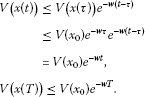When $T\le t,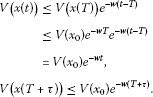When $T+\tau \le t<2T$,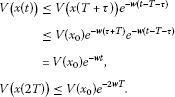When $2T\le t<2T+\tau$,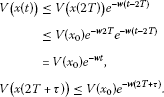When $nT\le t,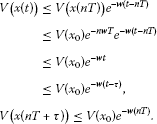(3.8)

When $nT+\tau \le t<\left(n+1\right)T$,

$\begin{array}{rcl}V\left(x\left(t\right)\right)& \le & V\left(x\left(nT+\tau \right)\right){e}^{-w\left(t-nT-\tau \right)}\\ \le & V\left({x}_{0}\right){e}^{-wnT}{e}^{-w\left(t-nT-\tau \right)}\\ =& V\left({x}_{0}\right){e}^{-w\left(t-\tau \right)}.\end{array}$
(3.9)

From (3.8) and (3.9), it follows that for any $t>0$, we can obtain

$V\left(x\left(t\right)\right)\le V\left({x}_{0}\right){e}^{-w\left(t-\tau \right)},\phantom{\rule{1em}{0ex}}\mathrm{\forall }t>0.$
(3.10)

From (3.3) and (3.10), it follows that for any $t>0$,

$\begin{array}{rcl}{x}^{T}\left(t\right)x\left(t\right)& \le & \frac{V\left(x\left(t\right)\right)}{{\lambda }_{min}\left(P\right)}\\ \le & \frac{1}{{\lambda }_{min}\left(P\right)}V\left({x}_{0}\right){e}^{-w\left(t-\tau \right)}.\end{array}$

Hence, we get

$\parallel x\left(t\right)\parallel \le \sqrt{\frac{V\left({x}_{0}\right)}{{\lambda }_{min}\left(P\right)}}{e}^{-\frac{w\left(t-\tau \right)}{2}},\phantom{\rule{1em}{0ex}}\mathrm{\forall }t>0,$

Noticing

$\begin{array}{rcl}V\left({x}_{0}\right)& =& {x}^{T}\left(0\right)Px\left(0\right)+{\int }_{-d}^{0}{e}^{w\theta }{x}^{T}\left(\theta \right)Qx\left(\theta \right)\phantom{\rule{0.2em}{0ex}}d\theta \\ \le & \left({\lambda }_{max}\left(P\right)+d{\lambda }_{max}\left(Q\right)\right){\parallel \varphi \parallel }^{2},\end{array}$

we get

$\parallel x\left(t\right)\parallel \le \parallel \varphi \parallel \sqrt{\frac{{\lambda }_{max}\left(P\right)+d{\lambda }_{max}\left(Q\right)}{{\lambda }_{min}\left(P\right)}}{e}^{-\frac{w\left(t-\tau \right)}{2}},\phantom{\rule{1em}{0ex}}\mathrm{\forall }t>0,$

which completes the proof. □

Corollary 3.1 If there exist positive definite matrices $P>0$, $Q>0$, and scalar constants ${\epsilon }_{j}>0$ ($j=1,2,3,4$), $w>0$, such that the following LMIs hold: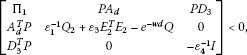(3.11)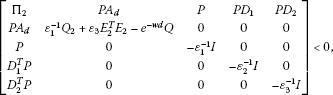(3.12)

were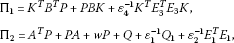then the system (2.4) is exponentially stable. Moreover, the solution $x\left(t\right)$ satisfies the condition

$\parallel x\left(t\right)\parallel \le \parallel \varphi \parallel \sqrt{\frac{{\lambda }_{max}\left(P\right)+d{\lambda }_{max}\left(Q\right)}{{\lambda }_{min}\left(P\right)}}{e}^{-\frac{w\left(t-\tau \right)}{2}},\phantom{\rule{1em}{0ex}}\mathrm{\forall }t>0.$

Proof Set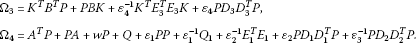According to Lemma 2.3 and (3.11) and (3.12), we have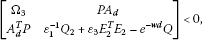(3.13)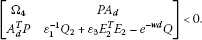(3.14)

Taking ${\epsilon }_{11}={\epsilon }_{21}={\epsilon }_{1}$, ${\epsilon }_{12}={\epsilon }_{22}={\epsilon }_{2}$, ${\epsilon }_{13}={\epsilon }_{23}={\epsilon }_{3}$, ${\epsilon }_{14}={\epsilon }_{4}$, we have

$\begin{array}{rcl}{\mathrm{\Omega }}_{2}& =& {A}^{T}P+PA+wP+Q+{\epsilon }_{21}PP+{\epsilon }_{21}^{-1}{Q}_{1}+{\epsilon }_{22}^{-1}{E}_{1}^{T}{E}_{1}+{\epsilon }_{22}P{D}_{1}{D}_{1}^{T}P+{\epsilon }_{23}^{-1}P{D}_{2}{D}_{2}^{T}P\\ =& {A}^{T}P+PA+wP+Q+{\epsilon }_{1}PP+{\epsilon }_{1}^{-1}{Q}_{1}+{\epsilon }_{2}^{-1}{E}_{1}^{T}{E}_{1}+{\epsilon }_{2}P{D}_{1}{D}_{1}^{T}P+{\epsilon }_{3}^{-1}P{D}_{2}{D}_{2}^{T}P\\ =& {\mathrm{\Omega }}_{4},\end{array}$

Hence, (3.14) implies that (3.2) holds and ${\mathrm{\Omega }}_{4}<0$. From (3.13), we have

$\left[\begin{array}{cc}{\mathrm{\Omega }}_{3}& P{A}_{d}\\ {A}_{d}^{T}P& {\epsilon }_{1}^{-1}{Q}_{2}+{\epsilon }_{3}{E}_{2}^{T}{E}_{2}-{e}^{-wd}Q\end{array}\right]+\left[\begin{array}{cc}{\mathrm{\Omega }}_{4}& 0\\ 0& 0\end{array}\right]<0.$
(3.15)

Since

$\begin{array}{rcl}{\mathrm{\Omega }}_{1}& =& {A}^{T}P+PA+{K}^{T}{B}^{T}P+PBK+wP+Q+{\epsilon }_{11}PP+{\epsilon }_{11}^{-1}{Q}_{1}+{\epsilon }_{12}^{-1}{E}_{1}^{T}{E}_{1}+{\epsilon }_{12}P{D}_{1}{D}_{1}^{T}P\\ +{\epsilon }_{14}^{-1}{K}^{T}{E}_{3}^{T}{E}_{3}K+{\epsilon }_{14}P{D}_{3}{D}_{3}^{T}P+{\epsilon }_{13}^{-1}P{D}_{2}{D}_{2}^{T}P\\ =& {A}^{T}P+PA+{K}^{T}{B}^{T}P+PBK+wP+Q+{\epsilon }_{1}PP+{\epsilon }_{1}^{-1}{Q}_{1}+{\epsilon }_{2}^{-1}{E}_{1}^{T}{E}_{1}+{\epsilon }_{2}P{D}_{1}{D}_{1}^{T}P\\ +{\epsilon }_{4}^{-1}{K}^{T}{E}_{3}^{T}{E}_{3}K+{\epsilon }_{4}P{D}_{3}{D}_{3}^{T}P+{\epsilon }_{3}^{-1}P{D}_{2}{D}_{2}^{T}P\\ =& {\mathrm{\Omega }}_{4}+{K}^{T}{B}^{T}P+PBK+{\epsilon }_{4}^{-1}{K}^{T}{E}_{3}^{T}{E}_{3}K+{\epsilon }_{4}P{D}_{3}{D}_{3}^{T}P={\mathrm{\Omega }}_{4}+{\mathrm{\Omega }}_{3},\end{array}$

(3.15) implies that (3.1) holds. According to Theorem 3.1, the system (2.4) is exponentially stable, and

$\parallel x\left(t\right)\parallel \le \parallel \varphi \parallel \sqrt{\frac{{\lambda }_{max}\left(P\right)+d{\lambda }_{max}\left(Q\right)}{{\lambda }_{min}\left(P\right)}}{e}^{-\frac{w\left(t-\tau \right)}{2}},\phantom{\rule{1em}{0ex}}\mathrm{\forall }t>0,$

which completes the proof. □

The following theorem can be directly deduced from Theorem 3.1 by applying Lemma 2.3.

Theorem 3.2 If there exist positive definite matrices $P>0$, $Q>0$ and scalar constants ${\epsilon }_{ij}>0$ ($i=1,2$, $j=1,2,3$), ${\epsilon }_{14}>0$, $w>0$, such that the following LMIs hold: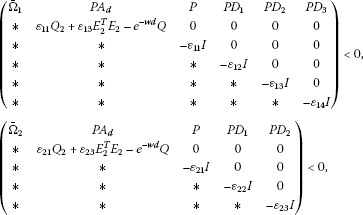where

${\overline{\mathrm{\Omega }}}_{1}={A}^{T}P+PA+{K}^{T}{B}^{T}P+PBK+wP+Q+{\epsilon }_{11}{Q}_{1}+{\epsilon }_{12}{E}_{1}^{T}{E}_{1}+{\epsilon }_{14}{K}^{T}{E}_{3}^{T}{E}_{3}K,$

and

${\overline{\mathrm{\Omega }}}_{2}={A}^{T}P+PA+wP+Q+{\epsilon }_{21}{Q}_{1}+{\epsilon }_{22}{E}_{1}^{T}{E}_{1},$

then the system (2.4) is exponentially stable. Moreover, the solution $x\left(t\right)$ satisfies the condition

$\parallel x\left(t\right)\parallel \le \parallel \varphi \parallel \sqrt{\frac{{\lambda }_{max}\left(P\right)+d{\lambda }_{max}\left(Q\right)}{{\lambda }_{min}\left(P\right)}}{e}^{-\frac{w\left(t-\tau \right)}{2}},\phantom{\rule{1em}{0ex}}\mathrm{\forall }t>0.$

Consider the following time-delay systems:

$\begin{array}{r}\stackrel{˙}{x}\left(t\right)=Ax\left(t\right)+{A}_{d}x\left(t-d\right)+Bu\left(t\right)+f\left(x\left(t\right),x\left(t-d\right)\right),\\ x\left(t\right)=\varphi \left(t\right),\phantom{\rule{1em}{0ex}}t\in \left[-d,0\right],\end{array}$
(3.16)

where $x\in {R}^{n}$ is a state vector, and $u\in {R}^{m}$ is the external input of the system (3.16). $f:{R}^{n}\to {R}^{n}$ is a continuous nonlinear function satisfying $f\left(0,0\right)=0$, and there exist positive definite matrices ${Q}_{1}$, ${Q}_{2}$ such that ${\parallel f\left(x\left(t\right),x\left(t-d\right)\right)\parallel }^{2}\le {x}^{T}\left(t\right){Q}_{1}x\left(t\right)+{x}^{T}\left(t-d\right){Q}_{2}x\left(t-d\right)$ for $x\left(t\right),x\left(t-d\right)\in {R}^{n}$.

With the control law (2.3), the system (3.16) can be rewritten as

$\left\{\begin{array}{cc}\stackrel{˙}{x}\left(t\right)=Ax\left(t\right)+{A}_{d}x\left(t-d\right)+BKx\left(t\right)+f\left(x\left(t\right),x\left(t-d\right)\right),\hfill & nT\le t
(3.17)

From Corollary 3.1, the following corollary can be immediately obtained.

Corollary 3.2 If there exist positive definite matrices $P>0$, $Q>0$, and scalar constants ${\epsilon }_{1}>0$, $w>0$, such that the following LMIs hold: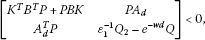(3.18)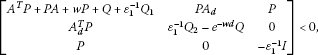(3.19)

then the system (3.17) is exponentially stable. Moreover, the solution $x\left(t\right)$ satisfies the condition

$\parallel x\left(t\right)\parallel \le \parallel \varphi \parallel \sqrt{\frac{{\lambda }_{max}\left(P\right)+d{\lambda }_{max}\left(Q\right)}{{\lambda }_{min}\left(P\right)}}{e}^{-\frac{w\left(t-\tau \right)}{2}},\phantom{\rule{1em}{0ex}}\mathrm{\forall }t>0.$

## 4 Numerical examples

The following numerical examples are presented to illustrate the usefulness of the proposed theoretical results in Section 3.

Example 1 Consider the following uncertain system:(4.1)

where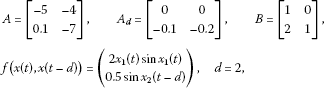and the uncertainties satisfy (2.2) with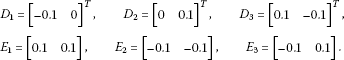It is easy to see that the nonlinearity of the system satisfies

$\begin{array}{rcl}{\parallel f\left(x\left(t\right),x\left(t-d\right)\right)\parallel }^{2}& =& {\left(2{x}_{1}\left(t\right)sin{x}_{1}\left(t\right)\right)}^{2}+0.25{sin}^{2}{x}_{2}\left(t-d\right)\\ \le & 4{x}_{1}^{2}\left(t\right)+0.25{x}_{2}^{2}\left(t-d\right).\end{array}$

Then there exist positive definite matrices

${Q}_{1}=\left[\begin{array}{cc}4& 0\\ 0& 0.1\end{array}\right],\phantom{\rule{2em}{0ex}}{Q}_{2}=\left[\begin{array}{cc}0.1& 0\\ 0& 0.25\end{array}\right],$

such that

Let ${\epsilon }_{1}={\epsilon }_{2}={\epsilon }_{3}={\epsilon }_{4}=1$, $w=0.5$ and $K=\left[\begin{array}{cc}-15& 8\\ 30& -30\end{array}\right]$ for convenience. Solving the inequalities (3.11) and (3.12), we get

$P=\left[\begin{array}{cc}0.7927& -0.3049\\ -0.3049& 0.4648\end{array}\right],\phantom{\rule{2em}{0ex}}Q=\left[\begin{array}{cc}2.0193& 0.0837\\ 0.0837& 2.6127\end{array}\right].$

Thus, according to Corollary 3.1, the system (4.1) is exponentially stabilizable with

$u\left(t\right)=\left\{\begin{array}{cc}\left[\begin{array}{cc}-15& 8\\ 30& -30\end{array}\right]x\left(t\right),\hfill & nT\le t

Example 2 Consider the following time-delay system:

$\begin{array}{r}\stackrel{˙}{x}\left(t\right)=Ax\left(t\right)+{A}_{d}x\left(t-d\right)+Bu\left(t\right)+f\left(x\left(t\right),x\left(t-d\right)\right),\\ x\left(t\right)=\varphi \left(t\right),\phantom{\rule{1em}{0ex}}t\in \left[-d,0\right],\end{array}$
(4.2)

where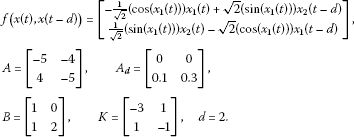We can verify that

${\parallel f\left(x\left(t\right),x\left(t-d\right)\right)\parallel }^{2}\le {x}^{T}\left(t\right){Q}_{1}x\left(t\right)+{x}^{T}\left(t-d\right){Q}_{2}x\left(t-d\right),$

where

${Q}_{1}=\left[\begin{array}{cc}1& 0\\ 0& 1\end{array}\right],\phantom{\rule{2em}{0ex}}{Q}_{2}=\left[\begin{array}{cc}2& 0\\ 0& 2\end{array}\right].$

For ${\epsilon }_{1}=1$, $w=0.5$, it is easy verify that

$P=\left[\begin{array}{cc}1.5& 0\\ 0& 1.5\end{array}\right],\phantom{\rule{2em}{0ex}}Q=\left[\begin{array}{cc}4e& 0\\ 0& 4e\end{array}\right],$

satisfy (3.18) and (3.19).

Hence, according to Corollary 3.2, the system (4.2) is exponentially stabilizable with

$u\left(t\right)=\left\{\begin{array}{cc}\left[\begin{array}{cc}-3& 1\\ 1& -1\end{array}\right]x\left(t\right),\hfill & nT\le t

## 5 Conclusions

In this brief, we deal with the exponential stabilization problem for a class of uncertain nonlinear systems with state delay by means of periodically intermittent control. Based on the Lyapunov-Krasovskii functional approach, several stability criteria have been presented in terms of a set of matrix inequalities. Moreover, the control method is extended to a class of nonlinear systems with time delay. A new sufficient condition, which guarantees the closed-loop system is exponentially stable, is presented. Finally, two numerical examples are provided to show the high performance of the proposed approach.

## References

1. Dong Y, Mei S, Wang X: Novel stability criteria of nonlinear uncertain systems with time-varying delay. Abstr. Appl. Anal. 2011., 2011: Article ID 969674. doi:10.1155/2011/969674

2. Phat VN, Hein LV: An application of Razumikhin theorem to exponential stability for linear non-autonomous systems with time-varying delay. Appl. Math. Lett. 2009, 22: 1412–1417. 10.1016/j.aml.2009.01.053

3. Dong Y, Liu J, Mei S, Li M: Stabilization for switched nonlinear time-delay systems. Nonlinear Anal. Hybrid Syst. 2011, 5: 78–88. 10.1016/j.nahs.2010.09.001

4. Bourkas E, Lin Z: Deterministic and Stochastic Time Delay systems. Birkhäuser, Boston; 2002.

5. Diblik J, Khusainov D, Khusarenko O: Representation of the solution for linear system of delay equations with distributed parameters. Nonlinear Dyn. Syst. Theory 2012, 13(2):251–268.

6. Khusainov DY, Diblik J, Bavstinec J, Ryvolova A: Interval stability of linear systems neutral type. Vychisl. Prikl. Mat. 2011, 3(106):148–160. in Russian

7. Dong Y, Wang X, Mei S, Li W: Exponential stabilization of nonlinear uncertain systems with time-varying delay. J. Eng. Math. 2012, 77: 225–237. 10.1007/s10665-012-9554-0

8. Shatyrko AV, Khusainov DY, Diblik J, Bavstinec J, Ryvolova A: Estimates of perturbation of nonlinear indirect interval control system of neutral type. J. Autom. Inf. Sci. 2011, 43: 13–28. 10.1615/JAutomatInfScien.v43.i1.20

9. Shatyrko AV, Khusainov DY: Absolute interval stability of indirect regulating systems of neutral type. J. Autom. Inf. Sci. 2010, 42: 43–54.

10. Shatyrko AV, Khusainov DY: Investigation of absolute stability of nonlinear systems of special kind with aftereffect by Lyapunov functions method. J. Autom. Inf. Sci. 2011, 43: 61–75.

11. Shatyrko AV, Khusainov DY: Absolute stability conditions construction of non-direct regulator systems by Lyapunov-Krasovskiy functional. Bull. Univ. Kiev, Ser. Phys. Math. 2009, 4: 145–152. in Ukrainian

12. Yue D: Robust stabilization of uncertain systems with unknown input delay. Automatica 2004, 40: 331–336. 10.1016/j.automatica.2003.10.005

13. Diblik J, Khusainov D, Lukavcova J, Raccuvzivckova M: Control of oscillating systems with a single delay. Adv. Differ. Equ. 2010., 2010: Article ID 108218. doi:10.1155/2010/108218

14. Diblik J, Khusainov DY, Grytsay IV, Šmarda Z: Stability of nonlinear autonomous quadratic discrete systems in the critical case. Discrete Dyn. Nat. Soc. 2010., 2010: Article ID 539087. doi:10.1155/2010/539087

15. Bavstinec J, Diblik J, Khusainov DY, Ryvolova A: Exponential stability and estimation of solutions of linear differential systems of neutral type with constant coefficients. Bound. Value Probl. 2010., 2010: Article ID 956121. doi:10.1155/2010/956121

16. Diblik J, Khusainov DY, Grytsay IV: Stability investigation of nonlinear quadratic discrete dynamics systems in the critical case. J. Phys. Conf. Ser. 2008., 96(1): Article ID 012042

17. Shatyrko AV, Khusainov DY: Interval stability investigations of differential regulator systems with time delay by Lyapunov-Krasovskiy functional. Bull. Univ. Kiev, Ser. Phys. Math. 2009, 3: 212–221. in Ukrainian

18. Dey R, Ray G, Ghosh S, Rakshit A: Stability analysis for continuous system with additive time-varying delay: a less conservative result. Appl. Math. Comput. 2010, 215: 3740–3745. 10.1016/j.amc.2009.11.014

19. Khusainov DY, Kojametov AT, Shatyrko AV: Interval stability of regulation systems with delay. Bull. Univ. Kiev, Ser. Phys. Math. 2001, 5: 73–88. in Ukrainian

20. Dzhalladova IA, Bastinec J, Diblik J, Khusainov DY: Estimates of exponential stability for solutions of stochastic control systems with delay. Abstr. Appl. Anal. 2011., 2011: Article ID 920412. doi:10.1155/2011/920412

21. Dong Y, Mei S: Global asymptotic stabilization of non-linear systems. Int. J. Control 2009, 82: 279–286. 10.1080/00207170802074486

22. Dong Y, Fan J, Mei S: Quadratic stabilization of switched nonlinear systems. Sci. China Ser. F: Inf. Sci. 2009, 52: 999–1006. 10.1007/s11432-009-0111-z

23. Lu JG, Hill DJ: Impulsive synchronization of chaotic Lur’e systems by linear static measurement feedback: an LMI approach. IEEE Trans. Circuits Syst. II, Express Briefs 2007, 54: 710–714.

24. Chen C, Ding Z, Feng G, Guan X: Output regulation of discrete-time nonlinear systems based on T-S fuzzy model. 27th Chinese Control Conference 2008, 746–750.

25. Montgomery TL, Frey JW, Norris WB: Intermittent control systems. Environ. Sci. Technol. 1975, 9: 528–532. 10.1021/es60104a608

26. Zochowski M: Intermittent dynamical control. Physica D, Nonlinear Phenom. 2000, 145: 181–190. 10.1016/S0167-2789(00)00112-3

27. Li CD, Liao XF, Huang TW: Exponential stabilization of chaotic systems with delay by periodically intermittent control. Chaos 2007., 17: Article ID 013103

28. Huang J, Li C, Han Q: Quasi-synchronization of chaotic neural networks with parameter mismatch by periodically intermittent control. 6. World Congress on Computer Science and Information Engineering 2009, 485–489.

29. Wang TY, Xie LH, Xie LH, De Souz CE: Robust control of a class of uncertain nonlinear systems. Syst. Control Lett. 1992, 19: 139–149. 10.1016/0167-6911(92)90097-C

30. Cao Y, Sun Y, Cheng C: Delay-dependent robust stabilization of uncertain systems with multiple state delays. IEEE Trans. Autom. Control 1998, 43: 1608–1612. 10.1109/9.728880

31. Kwon OM, Park JH: Exponential stability of uncertain dynamic systems including state delay. Appl. Math. Lett. 2006, 19: 901–907. 10.1016/j.aml.2005.10.017

## Acknowledgements

This work was supported by the Natural Science Foundation of Tianjin under Grant 11JCYBJC06800.

## Author information

Authors

### Corresponding author

Correspondence to Yali Dong.

### Competing interests

The authors declare that they have no competing interests.

### Authors’ contributions

YD carried out the main part of this manuscript. JL participated in the discussion and gave the examples. All authors read and approved the final manuscript.

## Rights and permissions

Open Access This article is distributed under the terms of the Creative Commons Attribution 2.0 International License (https://creativecommons.org/licenses/by/2.0), which permits unrestricted use, distribution, and reproduction in any medium, provided the original work is properly cited.

Reprints and Permissions

Dong, Y., Liu, J. Exponential stabilization of uncertain nonlinear time-delay systems. Adv Differ Equ 2012, 180 (2012). https://doi.org/10.1186/1687-1847-2012-180

• Accepted:

• Published:

• DOI: https://doi.org/10.1186/1687-1847-2012-180

### Keywords

• time-delay nonlinear systems
• periodically intermittent control
• exponential stabilization
• Lyapunov-Krasovskii functional
• linear matrix inequality (LMI)